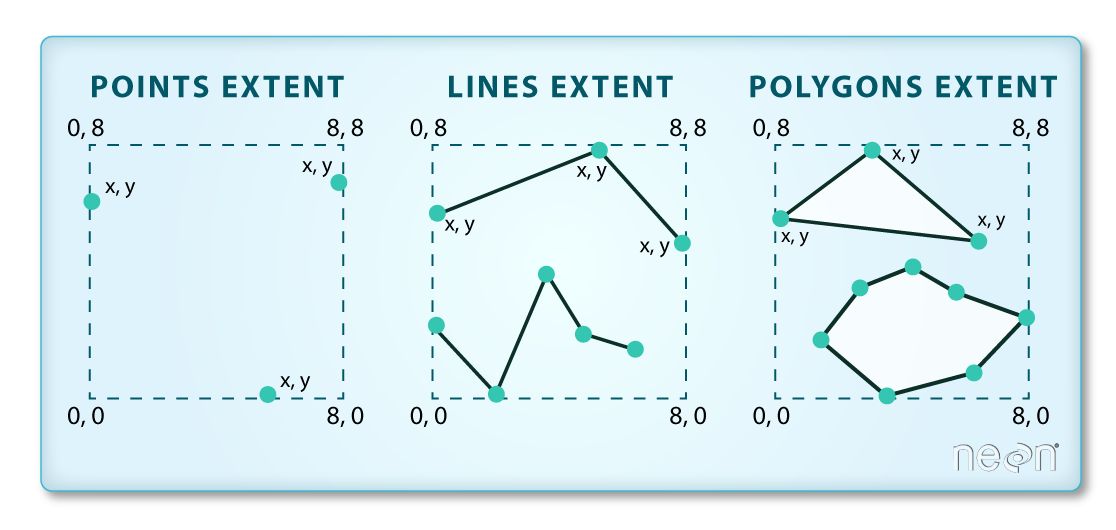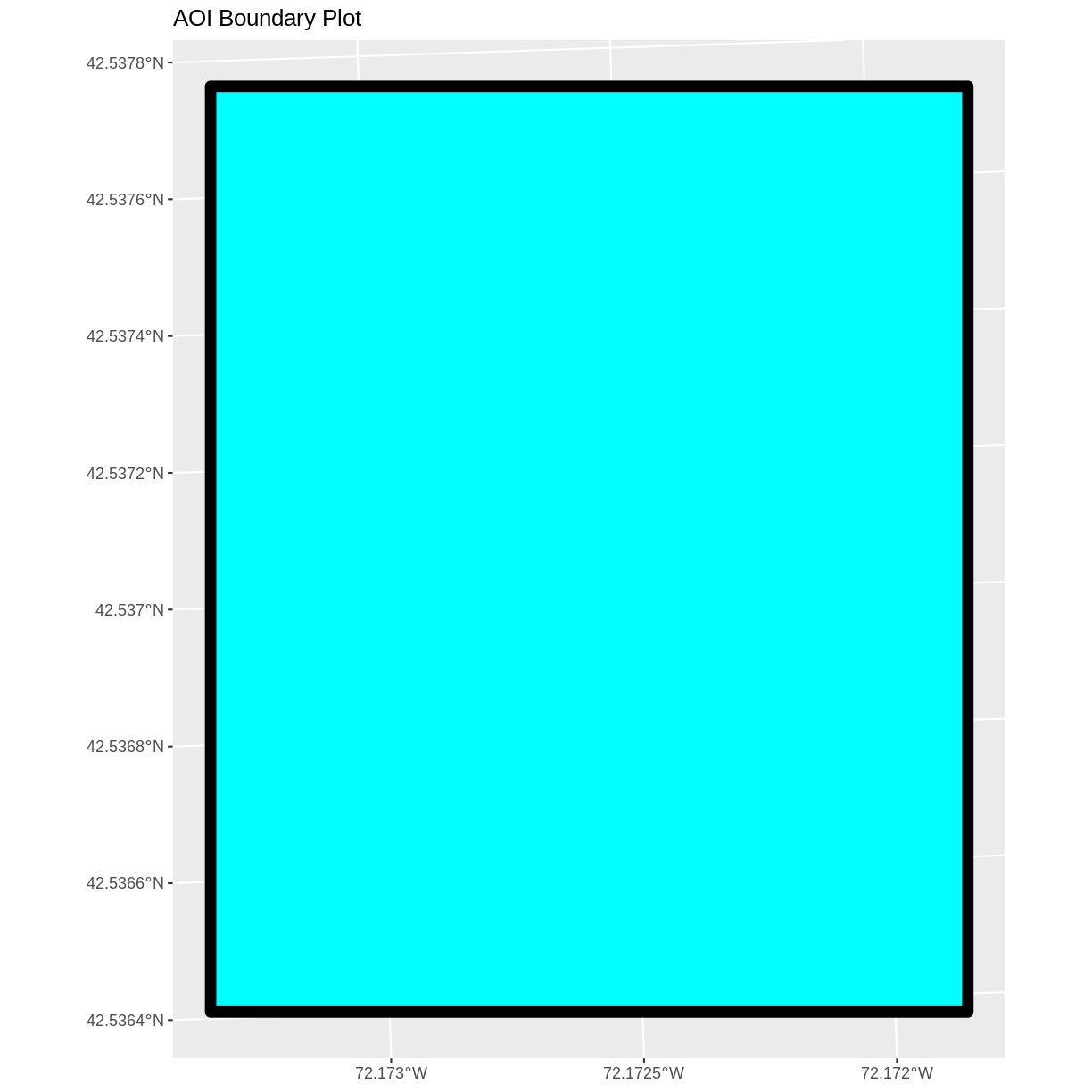This lesson is being piloted (Beta version)

# Open and Plot Shapefiles in R

## Overview

Teaching: 20 min
Exercises: 10 min
Questions
• How can I distinguish between and visualize point, line and polygon vector data?

Objectives
• Know the difference between point, line, and polygon vector elements.

• Load point, line, and polygon shapefiles into R.

• Access the attributes of a spatial object in R.

## Things You’ll Need To Complete This Episode

See the lesson homepage for detailed information about the software, data, and other prerequisites you will need to work through the examples in this episode.

Starting with this episode, we will be moving from working with raster data to working with vector data. In this episode, we will open and plot point, line and polygon vector data stored in shapefile format in R. These data refer to the NEON Harvard Forest field site, which we have been working with in previous episodes. In later episodes, we will learn how to work with raster and vector data together and combine them into a single plot.

## Import Shapefiles

We will use the `sf` package to work with vector data in R. Notice that the `rgdal` package automatically loads when `sf` is loaded. We will also use the `raster` package, which has been loaded in previous episodes, so we can explore raster and vector spatial metadata using similar commands. Make sure you have the `sf` library loaded.

``````library(sf)
``````

The shapefiles that we will import are:

The first shapefile that we will open contains the boundary of our study area (or our Area Of Interest or AOI, hence the name `aoiBoundary`). To import shapefiles we use the `sf` function `st_read()`. `st_read()` requires the file path to the shapefile.

Let’s import our AOI:

``````aoi_boundary_HARV <- st_read(
"data/vector/HarClip_UTMZ18.shp")
``````
``````Reading layer `HarClip_UTMZ18' from data source `/home/travis/build/UW-Madison-DataScience/r-raster-vector-geospatial/_episodes_rmd/data/vector/HarClip_UTMZ18.shp' using driver `ESRI Shapefile'
Simple feature collection with 1 feature and 1 field
geometry type:  POLYGON
dimension:      XY
bbox:           xmin: 732128 ymin: 4713209 xmax: 732251.1 ymax: 4713359
CRS:            32618
``````

When we import the `HarClip_UTMZ18` shapefile layer into R (as our `aoi_boundary_HARV` object), the `st_read()` function automatically stores information about the data. We are particularly interested in the geospatial metadata, describing the format, CRS, extent, and other components of the vector data, and the attributes which describe properties associated with each individual vector object.

## Data Tip

The Explore and Plot by Shapefile Attributes episode provides more information on both metadata and attributes and using attributes to subset and plot data.

Key metadata for all shapefiles include:

1. Object Type: the class of the imported object.
2. Coordinate Reference System (CRS): the projection of the data.
3. Extent: the spatial extent (i.e. geographic area that the shapefile covers) of the shapefile. Note that the spatial extent for a shapefile represents the combined extent for all spatial objects in the shapefile.

We can view shapefile metadata using the `st_geometry_type()`, `st_crs()` and `st_bbox()` functions. First, let’s view the geometry type for our AOI shapefile:

``````st_geometry_type(aoi_boundary_HARV)
``````
`````` POLYGON
18 Levels: GEOMETRY POINT LINESTRING POLYGON MULTIPOINT ... TRIANGLE
``````

Our `aoi_boundary_HARV` is a polygon object. The 18 levels shown below our output list the possible categories of the geometry type. Now let’s check what CRS this file data is in:

Remembering from an earlier lesson that CRS information uses a mathematical model to connect data to the Earth’s surface.

When we read in the shapefile into an R object, the metadata included the CRS – in this case is 32618 We can get more information about the CRS with the st_crs() function, and the proj4string method to get information on the CRS in the proj4string.

``````st_crs(aoi_boundary_HARV)\$proj4string
``````
`````` "+proj=utm +zone=18 +datum=WGS84 +units=m +no_defs "
``````

Our data in the CRS UTM zone 18N. The CRS is critical to interpreting the object’s extent values as it specifies units. To find the extent of our AOI, we can use the `st_bbox()` function:

``````st_bbox(aoi_boundary_HARV)
``````
``````     xmin      ymin      xmax      ymax
732128.0 4713208.7  732251.1 4713359.2
``````

The spatial extent of a shapefile or R spatial object represents the geographic “edge” or location that is the furthest north, south east and west. Thus is represents the overall geographic coverage of the spatial object. Image Source: National Ecological Observatory Network (NEON).Lastly, we can view all of the metadata and attributes for this shapefile object by printing it to the screen:

``````aoi_boundary_HARV
``````
``````Simple feature collection with 1 feature and 1 field
geometry type:  POLYGON
dimension:      XY
bbox:           xmin: 732128 ymin: 4713209 xmax: 732251.1 ymax: 4713359
CRS:            32618
id                       geometry
1  1 POLYGON ((732128 4713359, 7...
``````

## Spatial Data Attributes

We introduced the idea of spatial data attributes in an earlier lesson. Now we will explore how to use spatial data attributes stored in our data to plot different features.

## Plot a Shapefile

Next, let’s visualize the data in our `sf` object using the `ggplot` package. Unlike with raster data, we do not need to convert vector data to a dataframe before plotting with `ggplot`.

We’re going to customize our boundary plot by setting the size, color, and fill for our plot. When plotting `sf` objects with `ggplot2`, you need to use the `coord_sf()` coordinate system.

``````ggplot() +
geom_sf(data = aoi_boundary_HARV, size = 3, color = "black", fill = "cyan1") +
ggtitle("AOI Boundary Plot") +
coord_sf()
``````## Challenge: Import Line and Point Shapefiles

Using the steps above, import the HARV_roads and HARVtower_UTM18N layers into R. Call the HARV_roads object `lines_HARV` and the HARVtower_UTM18N `point_HARV`.

1. What type of R spatial object is created when you import each layer?

2. What is the CRS and extent for each object?

3. Do the files contain points, lines, or polygons?

4. How many spatial objects are in each file?

First we import the data:

``````lines_HARV <- st_read("data/vector/HARV_roads.shp")
``````
``````Reading layer `HARV_roads' from data source `/home/travis/build/UW-Madison-DataScience/r-raster-vector-geospatial/_episodes_rmd/data/vector/HARV_roads.shp' using driver `ESRI Shapefile'
Simple feature collection with 13 features and 15 fields
geometry type:  MULTILINESTRING
dimension:      XY
bbox:           xmin: 730741.2 ymin: 4711942 xmax: 733295.5 ymax: 4714260
CRS:            32618
``````
``````point_HARV <- st_read("data/vector/HARVtower_UTM18N.shp")
``````
``````Reading layer `HARVtower_UTM18N' from data source `/home/travis/build/UW-Madison-DataScience/r-raster-vector-geospatial/_episodes_rmd/data/vector/HARVtower_UTM18N.shp' using driver `ESRI Shapefile'
Simple feature collection with 1 feature and 14 fields
geometry type:  POINT
dimension:      XY
bbox:           xmin: 732183.2 ymin: 4713265 xmax: 732183.2 ymax: 4713265
CRS:            32618
``````

Then we check the geometry type of each object:

``````st_geometry_type(lines_HARV)
``````
``````  MULTILINESTRING MULTILINESTRING MULTILINESTRING MULTILINESTRING
 MULTILINESTRING MULTILINESTRING MULTILINESTRING MULTILINESTRING
 MULTILINESTRING MULTILINESTRING MULTILINESTRING MULTILINESTRING
 MULTILINESTRING
18 Levels: GEOMETRY POINT LINESTRING POLYGON MULTIPOINT ... TRIANGLE
``````
``````st_geometry_type(point_HARV)
``````
`````` POINT
18 Levels: GEOMETRY POINT LINESTRING POLYGON MULTIPOINT ... TRIANGLE
``````

We see that the lines_HARV has a number of multilinestring geometry items, and the point_HARV item has a point item.

We also check the CRS and extent of each object:

``````st_crs(lines_HARV)\$proj4string
``````
`````` "+proj=utm +zone=18 +datum=WGS84 +units=m +no_defs "
``````
``````st_bbox(lines_HARV)
``````
``````     xmin      ymin      xmax      ymax
730741.2 4711942.0  733295.5 4714260.0
``````
``````st_crs(point_HARV)\$proj4string
``````
`````` "+proj=utm +zone=18 +datum=WGS84 +units=m +no_defs "
``````
``````st_bbox(point_HARV)
``````
``````     xmin      ymin      xmax      ymax
732183.2 4713265.0  732183.2 4713265.0
``````

To see the number of objects in each file, we can look at the output from when we read these objects into R. `lines_HARV` contains 13 features (all lines) and `point_HARV` contains only one point.

## Key Points

• Shapefile metadata include geometry type, CRS, and extent.

• Load spatial objects into R with the `st_read()` function.

• Spatial objects can be plotted directly with `ggplot` using the `geom_sf()` function. No need to convert to a dataframe.